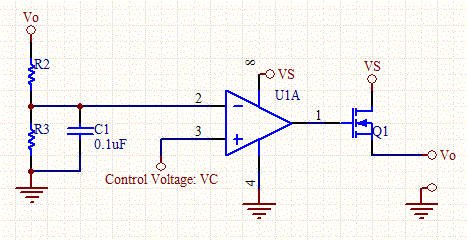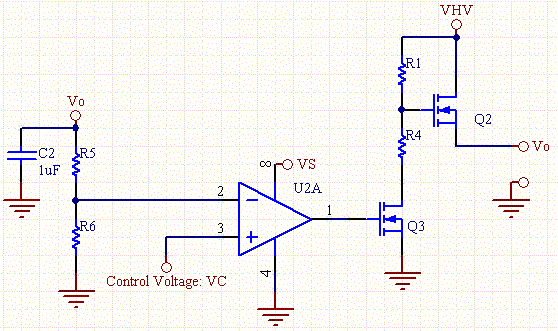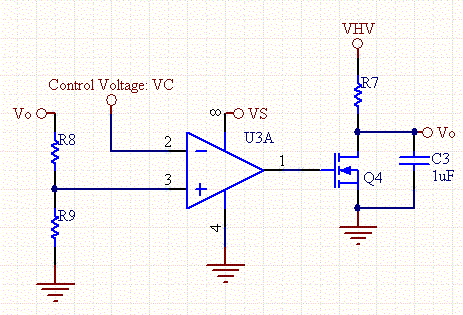# Voltage Controlled Voltage Source

This circuit allows a smaller control voltage to linearly control a larger output voltage Vo. The Resistors R2 and R3 scale the feedback to an appropriate range for the op-amp.

R3/(R2+R3)= VCmax/Vo

Note: VS>Vomax+Vgs(th).Voltage Controlled Voltage Source Schematic

The disadvantage of the circuit above is that the supply of the op-amp is tied to the HV supply. Most op-amps are limited to around 30V on the supply, so the circuit wont work for higher output voltages. The circuit below avoids this this problem by inserting a n-channel mosfet, which can be fully controlled within the range of the op-amps power supply.Voltage Controlled Voltage Source with N-Chan MOSFET Schematic

The output voltage is directly proportional to the control voltage. R1 and R4 should be chosen such that Vgs(max) of Q2 is never exceeded.Likewise Q2 should have an Idss greater than VHV.

Vo= (R5+R6)/R6*VC

Vgs(max)is typically around 20V for many MOSFETs. So the following should apply:

VHV*R4/(R1+R4)< Vgs(max)

The feedback resistors are set according to this equation:

R6/(R5+R6)= VCmax/Vo

Voltage Controlled Voltage Reference

The circuit below is a voltage controlled voltage reference. The output voltage is directly proportional to the control voltage.Note that the control voltage and feed back input of the op-amp is swapped.

Vo= (R8+R9)/R9*VC

The feedback resistors are set according to this equation:

R9/(R8+R9)= VCmax/Vo

The capacitor stops oscillation, and should only be placed on the output.The circuit will tend to oscillate if a capacitor is placed across the feedback network, for example across R9.Voltage Controlled Voltage Reference Schematic